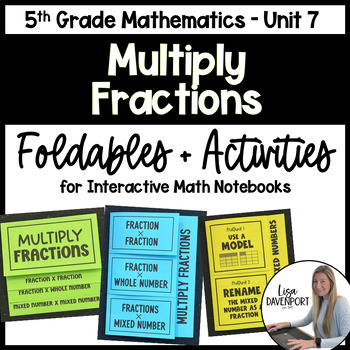# 5th Grade Math Foldable & Activity Bundle- Multiply FractionsSubject
Resource Type
Format
Zip (7 MB|4 foldable + 4 activities)
Standards
\$12.00
Bundle
List Price:
\$17.00
You Save:
\$5.00
Products in this Bundle (8)

showing 1-5 of 8 products

• Bundle Description
• Standards

PERFECT for interactive notebooks! These foldables work great during guided instruction, as a centers activity, collaborative assignment, exit ticket, or even a homework assignment.

An answer key is included with each product!

You may also be interested in some of my foldable bundles:

to see state-specific standards (only available in the US).
Solve real world problems involving multiplication of fractions and mixed numbers, e.g., by using visual fraction models or equations to represent the problem.
Explaining why multiplying a given number by a fraction greater than 1 results in a product greater than the given number (recognizing multiplication by whole numbers greater than 1 as a familiar case); explaining why multiplying a given number by a fraction less than 1 results in a product smaller than the given number; and relating the principle of fraction equivalence 𝘢/𝘣 = (𝘯×𝘢)/(𝘯×𝘣) to the effect of multiplying 𝘢/𝘣 by 1.
Comparing the size of a product to the size of one factor on the basis of the size of the other factor, without performing the indicated multiplication.
Interpret the product (𝘢/𝘣) × 𝘲 as a parts of a partition of 𝘲 into 𝘣 equal parts; equivalently, as the result of a sequence of operations 𝘢 × 𝘲 ÷ 𝘣. For example, use a visual fraction model to show (2/3) × 4 = 8/3, and create a story context for this equation. Do the same with (2/3) × (4/5) = 8/15. (In general, (𝘢/𝘣) × (𝘤/𝘥) = 𝘢𝘤/𝘣𝘥.)
Apply and extend previous understandings of multiplication to multiply a fraction or whole number by a fraction.
Total Pages
4 foldable + 4 activities
Included
Teaching Duration
N/A
Report this Resource to TpT
Reported resources will be reviewed by our team. Report this resource to let us know if this resource violates TpT’s content guidelines.
• Ratings & Reviews
• Q & A

Teachers Pay Teachers is an online marketplace where teachers buy and sell original educational materials.